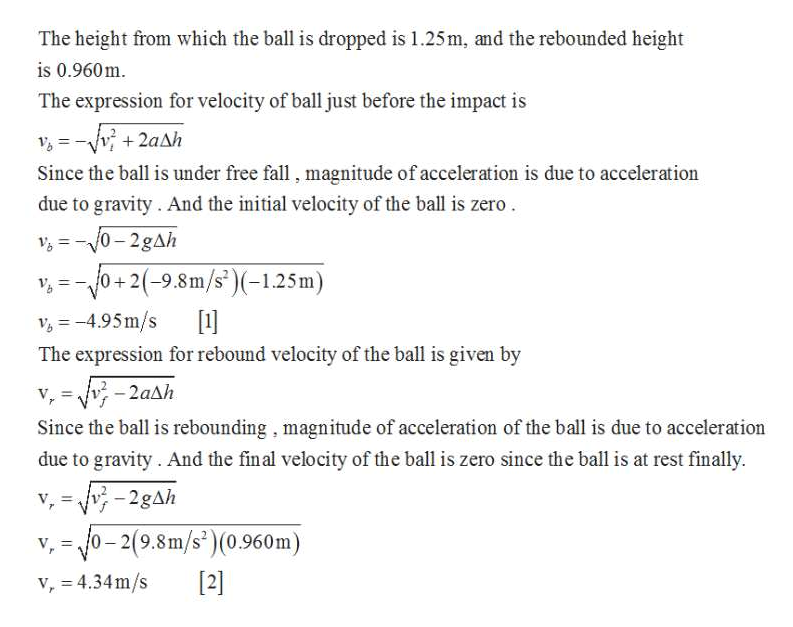# A ball of mass 0.150 kg is dropped from rest from a heightof 1.25 m. It rebounds from the floor to reach a height of0.960 m. What impulse was given to the ball by the floor?

Question
17 views

A ball of mass 0.150 kg is dropped from rest from a height
of 1.25 m. It rebounds from the floor to reach a height of
0.960 m. What impulse was given to the ball by the floor?

check_circle

Step 1help_outlineImage TranscriptioncloseThe height from which the ball is dropped is 1.25m, and the rebounded height is 0.960m. The expression for velocity of ball just before the impact is V = -Vv + 2aAh Since the ball is under free fall , magnitude of acceleration is due to acceleration due to gravity. And the initial velocity of the ball is zero. 0- 2gAh +2(-9.8m/s*)(-1.25m) v, = - 10 +20  V, = -4.95m/s The expression for rebound velocity of the ball is given by - 2aAh Since the ball is rebounding, magnitude of acceleration of the ball is due to acceleration due to gravity. And the final velocity of the ball is zero since the ball is at rest finally. v, = - 2gAh o- 2(9.8m/s*)(0.960m) V, %3D  v, = 4.34m/s %3D fullscreen

### Want to see the full answer?

See Solution

#### Want to see this answer and more?

Solutions are written by subject experts who are available 24/7. Questions are typically answered within 1 hour.*

See Solution
*Response times may vary by subject and question.
Tagged in

### Newtons Laws of Motion